Courses

# CAT Quant Mock Test - 2

## 25 Questions MCQ Test Quantitative Aptitude (Quant) | CAT Quant Mock Test - 2

Description
This mock test of CAT Quant Mock Test - 2 for CAT helps you for every CAT entrance exam. This contains 25 Multiple Choice Questions for CAT CAT Quant Mock Test - 2 (mcq) to study with solutions a complete question bank. The solved questions answers in this CAT Quant Mock Test - 2 quiz give you a good mix of easy questions and tough questions. CAT students definitely take this CAT Quant Mock Test - 2 exercise for a better result in the exam. You can find other CAT Quant Mock Test - 2 extra questions, long questions & short questions for CAT on EduRev as well by searching above.
QUESTION: 1

### DIRECTIONS for question: Select the correct alternative from the given choices. Find the ratio in which the line joining the points (-3, 3) and (2, 6) is divided by they-axis.

Solution: The ratio in which the y-axis divides the line joining (x1, y1) and (x2, y2) is – x1, : X2 = - (- 3): 2 = 3 : 2

QUESTION: 2

### DIRECTIONS for questions: Select the correct alternative from the given choices. There are 50 students divided into two groups, P and Q, with 20 and 30 students respectively. One student from group P shifts to group Q. If as a result of this, the average weight of group P decreases, while the average weight of group Q increases, which of the following holds true?

Solution:

Let the average weights of students in groups P and Q be p and q respectively. Total weights of students in groups P and Q are 20p and 30q respectively. Let the weight of the student who shifted from group P to group Q be w. After the shifting of the student,

the average weights of groups P and Q become 20p – w / 19 and 30q + w / 31respectively.

Given that20p – w / 19< p. Hence w > p.

Also given that 30q + w / 31> q. Hence w > q.

Alternative Solution

If a higher-average group joins or a lower-average group leaves, the average increases.

If a lower-average group joins or a higher-average group leaves, the average decreases.

The student leaves P and the average decreases. Therefore the student’s weight is more than initial (as well as the final) average weight of P. He joins Q and the average increases. Therefore, the student’s weight is more than the initial (as well as final) average weight of Q.

QUESTION: 3

### DIRECTIONS for questions: Select the correct alternative from the given choices. What is the least distance from the point (4, 0) to the curvex2 + y2 − 6y − 12x + 28 = 0?

Solution: x2 + y2 - 6y - 12x + 28 = 0

x2 - 12x + 36 + y2 - 6y + 9 + 28 = 36 + 9

(x - 6)2 + (y - 3)2 = 17

The above curve is a circle with centre at (6, 3) and radius √17 units. The least distance between the point and circle

= ∣Distance between the point and centre - Radius of the circle∣

Distance between the point (4, 0) and the center (6, 3) is√(6 – 4)2 + (3 – 0)2

= √22 + 32 = √13

=> least distance =√17 - √13 units.

QUESTION: 4

DIRECTIONS for questions: Select the correct alternative from the given choices.

During the placements in an MBA college, students were placed in four different sectors -Marketing (M), Finance (F), Operations (O) and IT (I). The averages of the annual CTCs of the students placed in M, F, O together and F, O, I together are Rs.13 lakh and Rs.14 lakh respectively, while the averages of the annual CTCs of the students placed in M, O, I together and M, F, I together are Rs.12 lakh and Rs.15 lakh respectively. Which of the following (in Rs. lakhs) could possibly be the average annual CTC of all the students placed?

I. 12.5

II. 13.6

III. 14

Solution: Let the number of students who get placed in Finance, Marketing, Operations and IT be f, m, o and i.

The average of the annual CTC’s of the students who are placed in M, F and O is 13.

=> The sum of the annual CTC’s of the students who are placed in M, F, O is 13(m + f + o) → (1)

Similarly, the sum of the annual CTC's of the students who are placed in F, O, I is 14(f + o + i) → (2)

The sum of the annual CTC’s of the students who are placed in M, O, I is 12(m + o + i) → (3)

The sum of the annual CTC’s of the students who are placed in M, F, I is 15(m + f + i) → (4}

We can observe that each of the four different sectors appears in exactly three of (1), (2), (3) and (4).

∴The average annual CTC of the students placed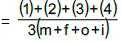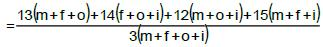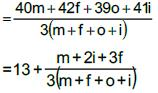Since, m + 2i + 3f will be less than 3 ( m + f + o + i), then average annual CTC of the students will be between 13 and 14.

∴Among the given options only II is possible.

QUESTION: 5

DIRECTIONS for questions: Select the correct alternative from the given choices.

A function f(x) is defined for a non-zero variable x, such that the value of f(y), where y = x + 1 / x, equals x2 + 1 / x2equals . The function f(x) can be

Solution:

Given that for a variable ‘x’, ‘f’ is such that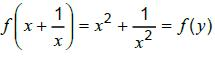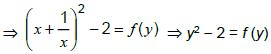Hence the function should be f(x) = x2 - 2

QUESTION: 6

DIRECTIONS for questions: Select the correct alternative from the given choices.

Five identical tennis balls are kept in a cylindrical container whose length is such that the five balls just fit into it in a single column. Find the ratio of the volume of the unoccupied portion of the container to that of the occupied portion, given that each ball is of the maximum possible size that can fit inside the container.

Solution: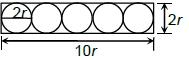The above figure gives the side view of the container and the balls.

Length of the box = 5 x 2r = 10r

Radius of the box = r

Volume of the box = πr2 x 10r = π10r3

Total volume of the five balls = 5 x 4/3 x π x r3 = 20r3π / 3

Unoccupied volume = 10πr3(1 – 2/3) = 10πr3(1 /3).

∴Required ratio = 10πr3: 20/3 πr3 =1:2

QUESTION: 7

DIRECTIONS for questions: Select the correct alternative from the given choices.

The arithmetic mean of a set of numbers was calculated as 24. However, the frequency of one of the numbers was erroneously taken as 6, instead of 9. As a result, the arithmetic mean calculated was 5 less than the actual mean. If all the numbers in the set are integers, then which of the following could be the sum of all frequencies?

Solution: Let the number whose frequency is taken erroneously be x.

Let the sum of frequencies initially considered be k

=> The actual sum of frequencies = k + 3

Calculated mean = 24

=> calculated sum of all observations = 24k

Actual sum = 24k + 3x

Actual mean = 24k + 3x / k + 3 = 24 + 5 = 29

=> 24k + 3x = 29k + 87

=> 5k = 3x - 87

=> k = 3(x – 29) / 5

As both k and x are integers, x - 29 must be a multiple of 5 and k must be a multiple of 3.

=> k + 3 must be a multiple of 3.

Among the given options, only 42 is a multiple of 3.

QUESTION: 8

DIRECTIONS for questions : Select the correct alternative from the given choices.

Find the sum of the first n terms of the series: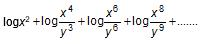Solution: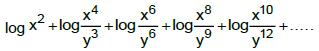2 logx + 4 logx - 3 logy + 6 logx - 6 logy + 8 logx - 9 logy + + 2n logx - 3(n - 1) logy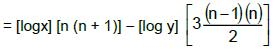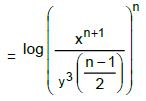Alternative Solution

This question can also be answered by eliminating the answer choices. By substituting n = 1, choices (b), (c) get eliminated and for n = 2; choice (d) is eliminated.

∴Only choice (a) can be the answer.

QUESTION: 9

DIRECTIONS for question: Select the correct alternative from the given choices.

WIMCO employs a total of 75 units to make coir products for export. The total monthly revenue from all the units is Rs.10,00,000. The total manufacturing cost and the total overheads (both of which vary with the number of units working) for all the units are Rs.1,50,000 and 1,00,000 every month respectively. If, in one month, the total profit reduced by Rs.35,000, how many units, on an average, were not working everyday during that month?

Solution: Total revenue = 10,00,000

Manufacturing Expenses = 1,50,000

Total Expenses = 2,50,000

Profit = 7,50,000

Profit/Unit = 10,000

Reduction in profit = 35,000 35,000

∴Average number of idle units = 35,000 / 10,000 =3.5

QUESTION: 10

DIRECTIONS for questions: Select the correct alternative from the given choices.

The breadth of a rectangle is decreased by 25%, as a result of which the perimeter of the rectangle decreased by 10%. By what percentage should the length of the rectangle now be increased, such that the perimeter of the rectangle will be the same as the initial one (i.e., before the breadth was decreased)?

Solution: Given, Let the length and breadth be ℓ and b respectively.

2(ℓ + 0.75b) = 0.9 x (2(ℓ + b))

=> ℓ + 0.75b = 0.9ℓ + 0.9b

=>0.1 ℓ = 0.15b

=>ℓ : b = 3 :2

Let ℓ = 30 and b = 20

=> Present ℓ, b = (30, 15), i.e., after decrease in breadth.

Now, the length should be increased by 5 in order to compensate (i.e., retain ℓ + b = 30 + 20 = 50) for the decrease in breadth.

Hence 5 / 30 x 100 = 16.67%

QUESTION: 11

DIRECTIONS for questions: Select the correct alternative from the given choices.

What is the radius (in cm) of the largest possible circle that can be cut out from a quadrant of a circle of radius 6 cm?

Solution: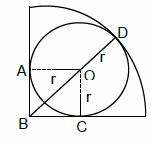The largest circle that can be cut from the quadrant must touch the two radii and the arc of the quadrant. Let its radius be r.

AOCB is a square where O is the centre of the circle to be cut.

= r√2 + r = 6

=> r = 6 / √2 + 1 = 6(√2 – 1)

Alternately, the radius of the smaller circle must be less than half the radius of the larger circle. Hence r < 3.="" by="" simple="" calculation,="" only="" choice="" (c)="" />

QUESTION: 12

DIRECTIONS for questions: Select the correct alternative from the given choices.

Find the positive square root of 13 + √48 + √72 + √96.

Solution: Let the required square root be √a + √b + √c

Now, (√a +√b +√c =a+b+c+2√ab +2√bc +2√ac

13+√48 + √72+ √96 = 13+2√12 + 2√18 + 2√24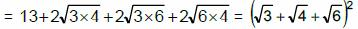Therefore the square root is

√a +√b +√c =√4+√3+√6

i.e. 2 + √3(√2+ 1)

Alternative Solution

Choices (A) and (C) can be easily eliminated since none of the surds in the given expression has √5 as factor. Choice (B) is also eliminated since √2x√8 would make the rational part much more (2 + 8 + 3 + 4= 17) than 13 (in the given expression). Hence, by elimination, Choice (d).

QUESTION: 13

DIRECTIONS for question: The question is followed by two statements, I and II. You have to decide whether the information provided in the statements is sufficient for answering the question and select the correct answer option.

Gayatri spent less than Rs.180 to buy three kg of each of rice, wheat and jowar. Which of the three cereals she bought was the cheapest?

I. 4 kg rice and 2 kg wheat cost less than 2 kg rice and 4 kg wheat.

II. 2 kg wheat and 4 kg jowar cost the same as 4 kg rice and 2 kg jowar.

Solution: Let the cost of 1 kg of rice, 1 kg of wheat and 1 kg of jowar be₹r, ₹w, ₹j respectively.

From I alone, we have

4r + 2w< 2r+4w

=> r < w.

It is not sufficient.

From n alone we have

2w + 4j = 4r + 2j => w+j= 2r

It is not sufficient

By combining both I and II, we can say that w> r> j.

QUESTION: 14

DIRECTIONS for questions: Select the correct alternative from the given choices. Robert bought an equal number of each of three kinds of articles, whose cost prices were Rs.8, Rs.25 and Rs.38 per article respectively. If, on the whole, he spent Rs.2,769, what is the total number of articles that he bought?

Solution: Price of each type of article is ₹8, ₹25, ₹38.

Let us assume Robert bought x articles of each kind.

Total Amount spent = 8x + 25x + 38x = 2769

=> 71x = 2769

=> x = 39

∴Robert bought 39 articles of each kind.

=>A total of 117 articles.

QUESTION: 15

DIRECTIONS for questions: Select the correct alternative from the given choices.

The range of cos6x + sin6x is

Solution: Let E = cos6x + sin6x

= (cos2x + sin2x)3 - 3 cos2x sin2x (cos2x + sin2x)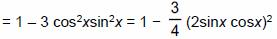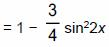For all x, 0 ≤ sin2 2x ≤ 1.

If sin2 2x = 0, then E = 1 and if sin2 2x= I, then E = 1 / 4

∴The required range is [1 / 4, 1]

Alternative Solution

Since sin6x + cos6x is always positive, choices (A) and (D) can be eliminated. Further, since both sinx and cosx cannot be simultaneously zero, even choice (C) is eliminated. Hence, choice (b).

QUESTION: 16

DIRECTIONS for questions: Select the correct alternative from the given choices.

A milkman has two containers, containing mixtures of milk and water. The first container has 110 litres of a mixture of milk and water in the ratio of 5 : 6. The second container has a mixture of milk and water in the ratio of 7 : 3. How much mixture should be taken from the second container and added to the first so that the ratio of the milk and water in the first container will become 6 : 5?

Solution: The quantity of milk in the first container = 50 litres

Quantity of water in the first container = 60 litre.

Let the quantity of mixture added from the second container be 10x. 50 + 7x : 60 + 3x = 6:5

=>x= 6.471

Hence, 10x= 64.71 litres of mixture must be added.

QUESTION: 17

DIRECTIONS for questions: Select the correct alternative from the given choices.

A man has a special rechargeable electric vehicle, which can maintain a speed ofx km/hr for (100 − x) minutes, after which it has to be recharged. What is the maximum distance (in km) that the man can cover on the vehicle without recharging in between?

Solution:

If the speed of x km/hr be maintained for 100 - x minutes, the maximum distance that 50 can be covered occurs when x = 100 -x, i.e., x - 50 and it is 50 / 60 (50) = 41 x 2 / 3km

QUESTION: 18

DIRECTIONS for question: Select the correct alternative from the given choices.

Ashok told his son, “x years ago, my age was twice your age and 5x years ago, my age was thrice your age.” If the difference of their present ages is 24 years, find the sum of their present ages (in years).

Solution: Let the present ages of Ashok and his son be A years and S years respectively.

Given A - S = 24

=> A = S + 24 — (1)

Also, x years ago (A- x) = 3(S - x)

=> (S + 24 - x) = 2(S - x)— (2)

Similarly, 5x years ago

(S + 24 - 5x) = 3(S - 5x) — (3)

Solving (2) and (3), x = 3, S = 27 and A = 51=> S + A = 78

Alternative solution

Since the difference A - S is even (i.e., 24) the sum also must be even. We could check for choice (B) and (D). Hence choices (1) and (3) are eliminated.

Further, for choice (B)

A + S = 78 and A - S = 24 => A = 51, S = 27

By some observation. (51 - 3) = 2(27 - 3) and (51 - 15)

= 3(27 - 15) i.e., x = 3

QUESTION: 19

At a parking lot, there are total of 50 two-wheelers and four-wheelers. The total number of wheels of these vehicles is 160. Find the number of two-wheelers at the parking lot.

Solution:

Let the numbers of two-wheelers and four-wheelers at the parking lot be T and F respectively

T + F = 50 and 2T + 4F = 160.

Solving these, T = 20 and F = 30.

QUESTION: 20

Find the length (in m) of the platform which a 600 m long train, travelling at 72 kmph, can cross in 50 seconds.

Solution:

The total distance travelled by train in 50 sec.

= 50 x 72 x 5 / 18 = 1000 m.

∴ The length of the platform = 1000 - 600 = 400 m

QUESTION: 21

A merchant purchased a pack of 15 bottles of soft drink, at a cost price of Rs.15 per bottle, and sold the pack at a profit of 20%. What is the additional profit (in Rs.) he would have made, if he had sold the pack at 15% more than what he sold it for?

[Enter your answer as a decimal value, rounded off to two decimal places.]

Solution: The total cost of buying the pack = 225

He sold it at 225 x 1.2 = 270

Profit he made = 270 - 225 = 45

If he sold it the next day, the selling price increased by 15% (the increase in C.P. would not affect the merchant since he has already purchased the pack before the price change)

Next dayS.P. = 270 x 1.15 = 310.5

Profit if he sold it the next day = 310.5 - 225 = 85.5

Additional profit = 85.5 - 45 = 40.5

QUESTION: 22

A work can be done by P in 48 days, whereas Q and R together can do the same work in 20 days. If Q is thrice as efficient as R, then P and R can do the work in

Solution: As Q is thrice as efficient as R, when they work together, they work with 4 times the efficiency of R. If only R works, he will take 4(20) = 80 days to complete the work.

∴ Time taken by P and R to complete the same work is (80)(48) / 80 + 48 = 30 days.

QUESTION: 23

This year, during the months of January and February, each day Ramu went to a fruit shop and bought exactly three varieties of fruits, such that for any two days, he bought at least one variety of fruit on one of the days that was different from what he bought on the other day. What is the minimum number of different varieties of fruits that he could have bought during that period?

Solution: Let the minimum number of varieties be n. Now, we have to select 3 fruits from these n. We have to choose n such that the number of selections (i.e., combinations of three out of n fruits) should be greater than (or) equal to the number of days, i.e., 31 + 28 = 59. i.e., nC3 ≥ 59

for n = 8 (8)(7)(6) / 6 = 56 < 59

So minimum possible n is 9.

QUESTION: 24

The following graph plots the time of travel (in seconds) and the speed of travel (in meters per second) of a particle. What is the total distance (in m) travelled by the particle from t = 0 to t = 4 seconds?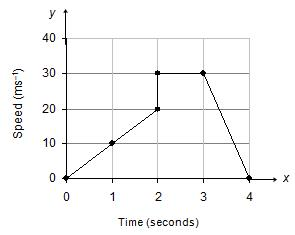Solution: The x-axis has time in secs and the y-axis has speed in mts. Hence the area of the plotted region will give us the distance travelled in m (not km). Area of the graph is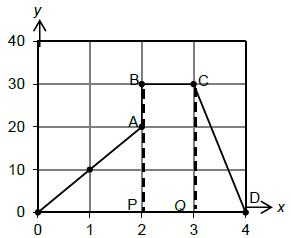= Area of AOAP + Area of rectangle PBCQ + Area of triangle QCD

= (1 / 2 x 2 x 20) + (1 x 30) + (1 / 2 x 1 x 30) = 20 + 30 + 15 = 65 m

∴ Distance travelled is 65 m

QUESTION: 25

If the area enclosed by the lines 3x − 2y + 6 = 0, 3x + 5y − 15 = 0 and the x-axis is A sq. units, find the value of 4A.

Solution: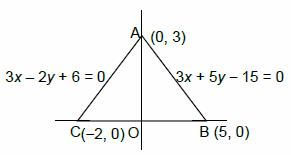The given lines 3x - 2y + 6 = 0 and 3x + 5y - 15 = 0 intersect on the y-axis at (0, 3). The area of the triangle formed by the lines 3x - 2y + 6 = 0, 3x + 5y - 15 = 0 and x-axis will be 1 / 2 x AO x BC = 1 / 2 x 3 x 7 = 10.5 sq.units. A = 10.5

∴4A =42